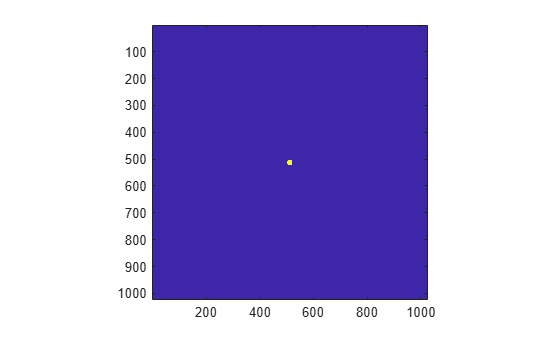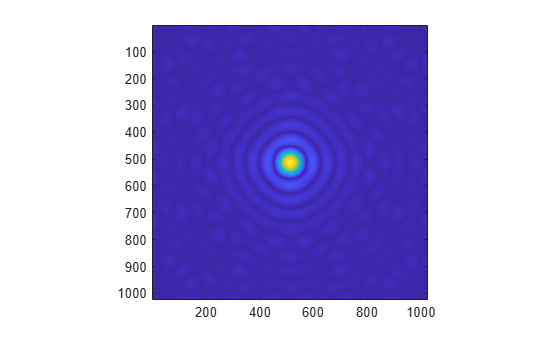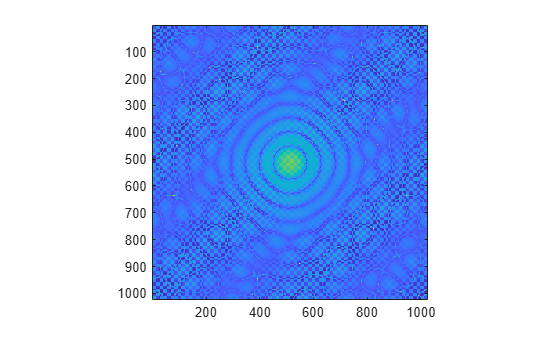Documentation

## 2-D Fourier Transforms

The `fft2` function transforms 2-D data into frequency space. For example, you can transform a 2-D optical mask to reveal its diffraction pattern.

### Two-Dimensional Fourier Transform

The following formula defines the discrete Fourier transform Y of an m-by-n matrix X.

`${Y}_{p+1,q+1}=\sum _{j=0}^{m-1}\sum _{k=0}^{n-1}{\omega }_{m}^{jp}{\omega }_{n}^{kq}{X}_{j+1,k+1}$`

ωm and ωn are complex roots of unity defined by the following equations.

`$\begin{array}{l}{\omega }_{m}={e}^{-2\pi i/m}\\ {\omega }_{n}={e}^{-2\pi i/n}\end{array}$`

i is the imaginary unit, p and j are indices that run from 0 to m–1, and q and k are indices that run from 0 to n–1. The indices for X and Y are shifted by 1 in this formula to reflect matrix indices in MATLAB®.

Computing the 2-D Fourier transform of X is equivalent to first computing the 1-D transform of each column of X, and then taking the 1-D transform of each row of the result. In other words, the command `fft2(X)` is equivalent to ```Y = fft(fft(X).').'```.

### 2-D Diffraction Pattern

In optics, the Fourier transform can be used to describe the diffraction pattern produced by a plane wave incident on an optical mask with a small aperture . This example uses the `fft2` function on an optical mask to compute its diffraction pattern.

Create a logical array that defines an optical mask with a small, circular aperture.

```n = 2^10; % size of mask M = zeros(n); I = 1:n; x = I-n/2; % mask x-coordinates y = n/2-I; % mask y-coordinates [X,Y] = meshgrid(x,y); % create 2-D mask grid R = 10; % aperture radius A = (X.^2 + Y.^2 <= R^2); % circular aperture of radius R M(A) = 1; % set mask elements inside aperture to 1 imagesc(M) % plot mask axis image```Use `fft2` to compute the 2-D Fourier transform of the mask, and use the `fftshift` function to rearrange the output so that the zero-frequency component is at the center. Plot the resulting diffraction pattern frequencies. Blue indicates small amplitudes and yellow indicates large amplitudes.

```DP = fftshift(fft2(M)); imagesc(abs(DP)) axis image```To enhance the details of regions with small amplitudes, plot the 2-D logarithm of the diffraction pattern. Very small amplitudes are affected by numerical round-off error, and the rectangular grid causes radial asymmetry.

```imagesc(abs(log2(DP))) axis image```Fowles, G. R. Introduction to Modern Optics. New York: Dover, 1989.